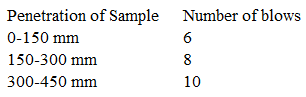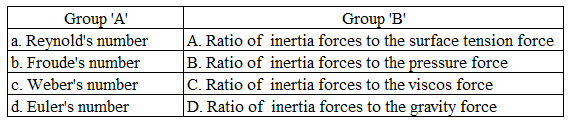# ISRO Scientist or Engineer Civil 2014

Instructions

For the following questions answer them individually

Question 11

# The modulus of Rupture of concrete in terms ofits characteristic cube compressive strength $$f_{ck}$$ in Mpa according to 1S456 — 2000 isQuestion 12

# For limit state of collapses, the partial safety factor recommended by IS456 — 2000 for estimating the design strength of concrete and reinforcement steel are respectivelyQuestion 13

# Creep Strain isQuestion 14

# Six columnsofbuilding are to be located within the plot size of 15x10m. The expected load on each column is 600 KN. Allowable bearing capacity of soil is 120 $$\frac{KN}{m^{2}}$$The type of foundation to be used isQuestion 15

# A clay soil sample is tested in tri-axial apparatus in consolidated drained conditions at a cell pressure of $$\frac{100 KN}{m^{2}}$$ What will be the pore water pressure at a deviator stress of $$\frac{40 KN}{m^{2}}$$Question 16

# The number of blows observed in a standard penetration test (SPT) for different depth are given below:The observed ‘N’ value isQuestion 17

# A sampling tube has an inner diameter of 80 mm and outer diameter of 88 mm. The area ratio isQuestion 18

# A compacted sample of soil with bulk density of $$2.0 g/cm^{3}$$ has a water content of 15%. What is its dry density? Assume G = 2.65Question 19

# A wet sample of sand weighing 415g is taken for water content determination by a pyconometer. The mass of pyconometer, sand and water is 1667g and mass of pyconometer full of water alone is 1440g.Assume G = 2.67. Determine water content.Question 20

# Match the Dimensionless number in Group ‘A’ to their definitions in Group ‘B’.OR